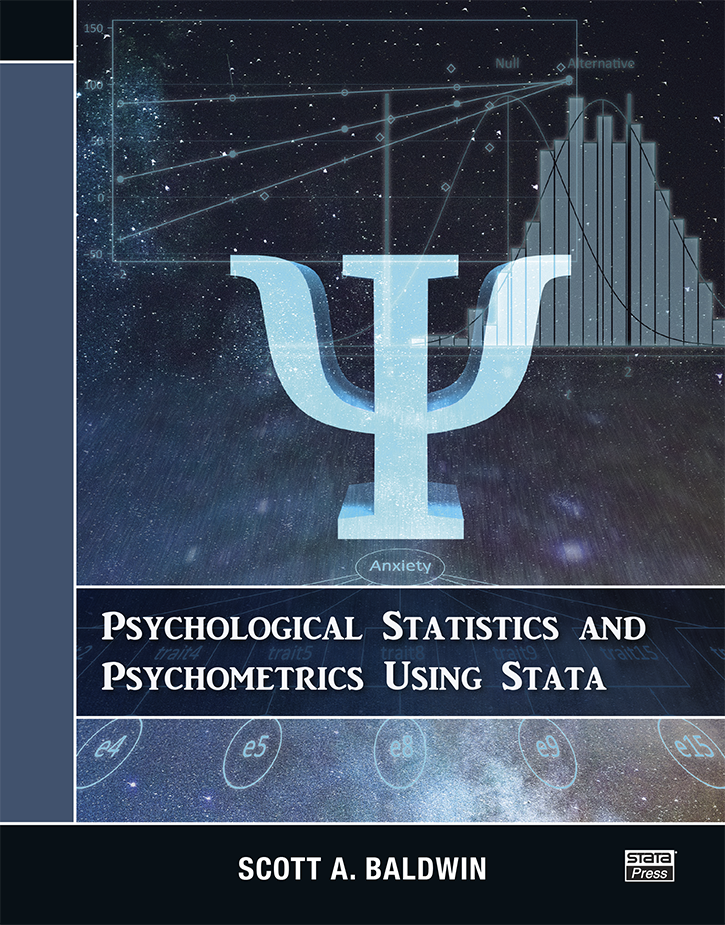# Psychological Statistics and Psychometrics Using Stata

Psychological Statistics and Psychometrics Using Stata by Scott Baldwin is a complete and concise resource for students and researchers in the behavioral sciences.

Baldwin’s primary goal in this book is to help readers become competent users of statistics. To that end, he first introduces basic statistical methods such as regression, t tests, and ANOVA. He focuses on explaining the models, how they can be used with different types of variables, and how to interpret the results. After building this foundation, Baldwin covers more advanced statistical techniques, including power-and-sample size calculations, multilevel modeling, and structural equation modeling. This book also discusses measurement concepts that are crucial in psychometrics. For instance, Baldwin explores how reliability and validity can be understood and evaluated using exploratory and confirmatory factor analysis. Baldwin includes dozens of worked examples using real data to illustrate the theory and concepts.

In addition to teaching statistical topics, this book helps readers become proficient Stata users. Baldwin teaches Stata basics ranging from navigating the interface to using features for data management, descriptive statistics, and graphics. He emphasizes the need for reproducibility in data analysis; therefore, he is careful to explain how version control and do-files can be used to ensure that results are reproducible. As each statistical concept is introduced, the corresponding commands for fitting and interpreting models are demonstrated. Beyond this, readers learn how to run simulations in Stata to help them better understand the models they are fitting and other statistical concepts.

This book is an excellent textbook for graduate-level courses in psychometrics. It is also an ideal reference for psychometricians and other social scientists who are new to Stata.

List of figures
List of tables
Acknowledgments
Notation and Typography

Getting oriented to Stata

1. INTRODUCTION

Structure of the book
Benefits of Stata
Scientific context

2. INTRODUCTION TO STATA

Point-and-click versus writing commands
The Stata interface
Getting data in Stata
Viewing and desribing data

list, in, and if

Creating new variables

Missing data
Labels

Summarizing data

summarize
table and tabulate

Graphing data

Histograms
Box plots
Scatterplots

Reproducible analysis

Do-files
Log files
Project Manager
Workflow

Getting help

Help documents
PDF documentation

Extending Stata

Statistical Software Components

Understanding relationships between variables

3. REGRESSION WITH CONTINUOUS PREDICTORS

Data
Exploration

Demonstration

Simulation program

Bivariate regression

Lines
Regression equation
Estimation
Interpretation
Slope
Intercept
Residuals and predicted values
Partitioning variance
Confidence intervals
Null hypothesis significance testing
Using predicted scores to understand model implications
Composite contrasts

Conclusions

4 REGRESSION WITH CATEGORICAL AND CONTINUOS PREDICTORS

Data for this chapter
Why categorical predictors need special care
Dummy coding

Example: Incorrect use of categorical variable

Multiple predictors

Interpretation
Model fit
Intercept
Slopes

Unique variance

Interactions

Categorical by continuous interactions
Dichotomous by continuous interactions
Polytomous by continuous interactions
Joint test for interactions with polytomous variables

Continuous by continuous interactions

Summary

5 T TESTS AND ONE-WAY ANOVA

Data
Comparing two means

t test
Effect size

Comparing three or more means

Analysis of variance
Multiple comparisons

Planned comparisons

Summary

6 Factorial ANOVA

Data for this chapter
Factorial design with two factors

Examining and visualizing the data
Main effects
Testing the null hypothesis

Interactions
Partitioning the variance
2 x 2 source table
Using anova to estimate a factorial ANOVA
Simple effects
Effect size

Factorial design with three factors

Examining and visualizing the data
Marginal means
Main effects and interactions
Three-way interaction
Fitting the model with anova
Interpreting the interaction

Conclusion

7 REPEATED-mEASURES MODELS

Data for this chapter
Basic model
Using mixed to fit a repeated-measures model

Covariance structures
Compound symmetry (exchangeable)
First-order autoregressive
Toeplitz
Unstructures

Degrees of freedom
Pairwise comparisons
Models with multiple factors
Estimating heteroskedastic residuals
Summary

8 PLANNING STUDIES: POWER AND SAMPLE- SIZE CALCULATIONS

Foundational ideas

Null and alternative distributions
Simulating draws out of the null and alternative distributions

Computing power manually
Stata’s commands

Two-sample z test
Two-sample t test
Correlation
One-way ANOVA
Factorial ANOVA

The central importance of power

Type M and S errors
Type S errors

Summary

9 MULTILEVEL MODELS FOR CROSS-SECTIONAL DATA

Data used in this chapter
Why clustered data structures matter

Statistical issues
Conceptual issues

Basics of a multilevel model

Partitioning sources of variance
Random intercepts
Estimating random intercepts
Intraclass correlations
Estimating cluster means
Comparing pooled and unpooled means

Between-clusters and within-cluster relationships

Partitioning variance in the predictor
Total- versus level-specific relationships
Exploring the between-clusters and within-cluster relationships
Estimating the between-clusters and within-cluster effects

Random slopes
Summary

10 MULTILEVEL MODELS FOR LONGITUDINAL DATA

Data used in this chapter
Basic growth model

Multilevel model

Summary
Psychometrics through the lens of factor analysis

11 FACTOR ANALYSIS: RELIABILITY

What you will learn in this chapter
Example data
Common versus unique variance
One-factor model

Parts of a path model
Where do the latent variables come from?

Prediction equation
Using sem to estimate CFA models
Model fit

Computing χ²
Obtaining σ²C and σ²U
Computing R² for an item
Computing σ²C and σ²U for all items
Computing reliability—ω
Bootstrapping the standard error and 95% confidence interval for ω

Comparing ω with α

Evaluating the assumption of tau-equivalence
Parallel items
Correlated residuals

Summary

12. FACTOR ANALYSIS: FACTORIAL VALIDITY

Data for this chapter
Exploratory factor analysis

Common factor model

Extraction methods
Eigenvalues
Communality and uniqueness
Factor analysis versus principal-component analysis
Choosing factors and rotation
How many factors should we extract?
Eigenvalue-greater-than-one rule
Scree plots
Parallel analysis
Orthogonal rotation—varimax
Oblique rotation—promax

Confirmatory factor analysis

EFA versus CFA
Estimating a CFA with sem
Mean structure versus variance structure
Identifying models
Imposing constraints for identification
How much information is needed to identify a model?
Refitting the model with constrained latent variables
Standardized solutions
Global fit
RMSEA
TLI
CFI
SRMR
A summary and a caution
Refining models further
Parallel items
Summary
Measurement invariance
Data
Measurement invariance
Measurement invariance across groups

Configural invariance
Metric invariance
Scalar invariance
Residual invariance
Using the comparative fit index to evaluate invariance

Structural invariance
Invariant factor variances
Invariant factor means
Measurement invariance across time

Configural invariance
Effects coding for identification
Effects-coding constraints in Stata
Metric invariance
Scalar invariance
Residual invariance

Structural invariance
Summary
References
Author index
Subject indexAuthor: Scott Baldwin
ISBN-13: 978-1-59718-303-1